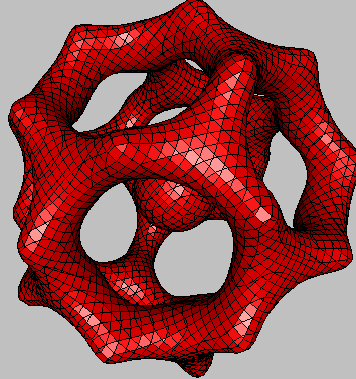Author Message
nextstep

Joined: 06 Jan 2007
Posts: 539Posted: Wed Jan 10, 2007 6:12 am    Post subject: IcosahedronHi all,
One beautiful example with it's associated minimal .Obj mesh generated
by K3DSurf:
 Quote: F(x, y, z) = if((x^2 + y^2 +z^2 < 35), 2 - (cos(x + (1+sqrt(5))/2*y) + cos(x - (1+sqrt(5))/2*y) + cos(y + (1+sqrt(5))/2*z) + cos(y - (1+sqrt(5))/2*z) + cos(z - (1+sqrt(5))/2*x) + cos(z + (1+sqrt(5))/2*x)) , 1) [x]:-5.5, 5.5 [y]:-5.5, 5.5 [z]:-5.5, 5.5

( and Yes, formulas with the "if" instruction
should work with all K3DSurf version)_________________
Cheers,
AbderrahmaninodeJoined: 27 Jan 2007
Posts: 127
Location: AustriaPosted: Fri Feb 09, 2007 2:56 pm    Post subject:Hi all

This figure can be also defined without a condition:
 Code: F(x,y,z) = 2 - (cos(x + (1+sqrt(5))/2*y) + cos(x - (1+sqrt(5))/2*y) + cos(y + (1+sqrt(5))/2*z) + cos(y - (1+sqrt(5))/2*z) + cos(z - (1+sqrt(5))/2*x) + cos(z + (1+sqrt(5))/2*x))*16/(x*x+y*y+z*z) [x]: -5, 5 [y]: -5, 5 [z]: -5, 5And if you want a different color for the sphere inside,
activate CND with the formula (x^2+y^2+z^2 > 3)if you choose (x^2+y^2+z^2 > 2.5)
you'll get patterned sphere.

Last edited by inode on Sun Feb 25, 2007 8:10 am; edited 3 times in totalnextstep

Joined: 06 Jan 2007
Posts: 539Posted: Fri Feb 09, 2007 3:54 pm    Post subject:Hi all, Brillant idea inode._________________Cheers, Abderrahmantomot

Joined: 21 Jul 2007
Posts: 23
Location: VancouverPosted: Sat Apr 04, 2009 12:58 am    Post subject:FYI: To make the Icosahedron you have to use the following code, if you are using the latest version of K3dSurf:

 Code: F():if((x^2 + y^2 +z^2 < 35), 2 - (cos(x + (1+sqrt(5))/2*y) + cos(x - (1+sqrt(5))/2*y) + cos(y + (1+sqrt(5))/2*z) + cos(y - (1+sqrt(5))/2*z) + cos(z - (1+sqrt(5))/2*x) + cos(z + (1+sqrt(5))/2*x)) , (x^2 + y^2 +z^2 -35)) [x]:-5.5, 5.5 [y]:-5.5, 5.5 [z]:-5.5, 5.5tomot

Joined: 21 Jul 2007
Posts: 23
Location: VancouverPosted: Sat Apr 11, 2009 5:18 pm    Post subject:OOPS! I did not notice it was an example in the main loaded file, sorry!Display posts from previous: All Posts1 Day7 Days2 Weeks1 Month3 Months6 Months1 Year Oldest FirstNewest First
 All times are GMT Page 1 of 1

 Jump to: Select a forum MathMod----------------MathMod Progress & featuresMathematical Models CollectionHow toBugsMathMod for WindowsMathMod for Linux/UnixMathMod For MACOSXMathMod TutorialsOther Mathematical ToolsOpen Discussion K3DSurf----------------K3DSurf Discuss/DiscussionsHow toK3DSurf math related questionsK3DSurf for WindowsK3DSurf for MacOSK3DSurf for LinuxOnline K3DSurf: J3DSurfNewsBugsOnline DocumentationAccount Activation Issue ?/ Problème d'activation de votre compte ?
You cannot post new topics in this forum
You cannot reply to topics in this forum
You cannot edit your posts in this forum
You cannot delete your posts in this forum
You cannot vote in polls in this forum